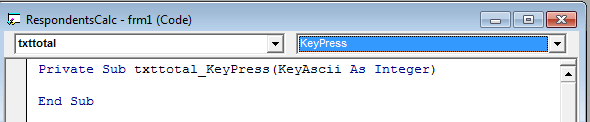# Compute Number of Respondents in Visual Basic

Problem:

Create a vb6 program that will ask the user to enter the total population then the program will compute the sample size.

Sample size – the number of respondents that will be the selected to answer the survey or questions.

Example: Total population = 1000, sample size = 100

Meaning out of 1000 only 100 will be part of the survey.

Solution:

To compute the sample size, we will use the slovin’s formula.

We can determine the number of respondents needed using this formula:

n = N / (1 + N e2)

Where:

n = is the sample size

N = is the total population

e = is the margin of error (0.05) usual value

Let’s start to code it

1. Open your visual basic 6 program then select Standard EXE.
2. A new project will be created with a Form1 on it.
3. We need to add the following control or object to our form:
1. 2 labels
2. 2 textboxes
3. 1 command button
4. This is how we design the form.Name the first textbox (with the label Enter Total number of Population) into txttotal.

The second textbox (with the label Respondents (sample size)) into txtsample.

1. Double click the txttotal textbox and select the keypress event.2. Paste the code to the keypress event ot txttotal.
```If (KeyAscii >= vbKey0 And KeyAscii <= vbKey9) Or KeyAscii = 46 Or KeyAscii = vbKeyBack Then
Else
Beep
KeyAscii = 0
End If
```

Code Explanation:

The code above will prevent the user to enter a non-numeric value to the textbox.

1. Double click again the txttotal and this time select the change event and paste this code.
```txtsample.Text = Val(txttotal.Text) / (1 + (Val(txttotal.Text) * 0.0025))
```

Code Explanation:

The code above will compute the sample size whenever the user has entered the population in the txttotal textbox.

1. To close the form double click the Close button and type the code
```Unload me
```
1. Save and Run the project.